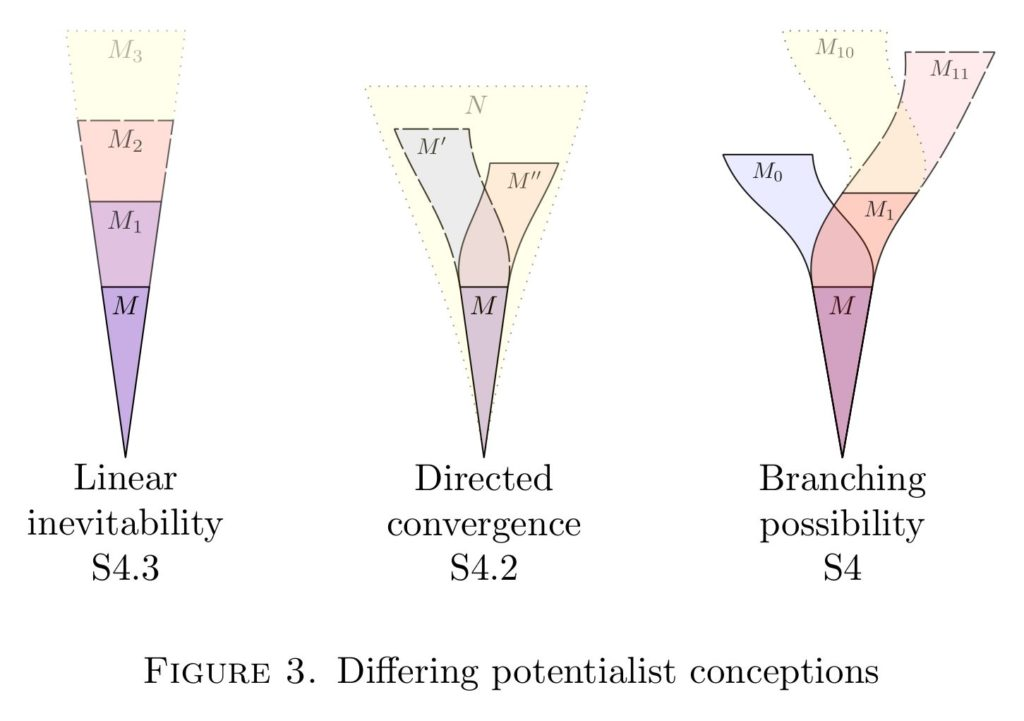# Modal model theory, STUK 4, Oxford, December 2019

This will be my talk for the Set Theory in the United Kingdom 4, a conference to be held in Oxford on 14 December 2019. I am organizing the conference with Sam Adam-Day.## Modal model theory

Abstract. I shall introduce the subject of modal model theory, a research effort bringing modal concepts and vocabulary into model theory. For any first-order theory T, we may naturally consider the models of T as a Kripke model under the submodel relation, and thereby naturally expand the language of T to include the modal operators. In the class of all graphs, for example, a statement is possible in a graph, if it is true in some larger graph, having that graph as an induced subgraph, and a statement is necessary when it is true in all such larger graphs. The modal expansion of the language is quite powerful: in graphs it can express k-colorability and even finiteness and countability. The main idea applies to any collection of models with an extension concept. The principal questions are: what are the modal validities exhibited by the class of models or by individual models? For example, a countable graph validates S5 for graph theoretic assertions with parameters, for example, just in case it is the countable random graph; and without parameters, just in case it is universal for all finite graphs. Similar results apply with digraphs, groups, fields and orders. This is joint work with Wojciech Wołoszyn.

Hand-written lecture notes

# The modal logic of arithmetic potentialism and the universal algorithm

• J. D. Hamkins, “The modal logic of arithmetic potentialism and the universal algorithm,” ArXiv e-prints, p. 1–35, 2018.
[Bibtex]
@ARTICLE{Hamkins:The-modal-logic-of-arithmetic-potentialism,
author = {Joel David Hamkins},
title = {The modal logic of arithmetic potentialism and the universal algorithm},
journal = {ArXiv e-prints},
year = {2018},
volume = {},
number = {},
pages = {1--35},
month = {},
eprint = {1801.04599},
archivePrefix = {arXiv},
primaryClass = {math.LO},
note = {Under review},
url = {http://wp.me/p5M0LV-1Dh},
abstract = {},
keywords = {under-review},
source = {},
doi = {},
}Abstract. Natural potentialist systems arise from the models of arithmetic when they are considered under their various natural extension concepts, such as end-extensions, arbitrary extension, $\Sigma_n$-elementary extensions, conservative extensions and more. For these potentialist systems, I prove, a propositional modal assertion is valid in a model of arithmetic, with respect to assertions in the language of arithmetic with parameters, exactly when it is an assertion of S4. Meanwhile, with respect to sentences, the validities of a model are always between S4 and S5, and these bounds are sharp in that both endpoints are realized. The models validating exactly S5 are the models of the arithmetic maximality principle, which asserts that every possibly necessary statement is already true, and these models are equivalently characterized as those satisfying a maximal $\Sigma_1$ theory. The main proof makes fundamental use of the universal algorithm, of which this article provides a self-contained account.

In this article, I consider the models of arithmetic under various natural extension concepts, including end-extensions, arbitrary extensions, $\Sigma_n$-elementary extensions, conservative extensions and more. Each extension concept gives rise to an arithmetic potentialist system, a Kripke model of possible arithmetic worlds, and the main goal is to discover the modal validities of these systems.For most of the extension concepts, a modal assertion is valid with respect to assertions in the language of arithmetic, allowing parameters, exactly when it is an assertion of the modal theory S4. For sentences, however, the modal validities form a theory between S4 and S5, with both endpoints being realized. A model of arithmetic validates S5 with respect to sentences just in case it is a model of the arithmetic maximality principle, and these models are equivalently characterized as those realizing a maximal $\Sigma_1$ theory.

The main argument relies fundamentally on the universal algorithm, the theorem due to Woodin that there is a Turing machine program that can enumerate any finite sequence in the right model of arithmetic, and furthermore this model can be end-extended so as to realize any further extension of that sequence available in the model. In the paper, I give a self-contained account of this theorem using my simplified proof.The paper concludes with philosophical remarks on the nature of potentialism, including a discussion of how the linear inevitability form of potentialism is actually much closer to actualism than the more radical forms of potentialism, which exhibit branching possibility. I also propose to view the philosphy of ultrafinitism in modal terms as a form of potentialism, pushing the issue of branching possibility in ultrafinitism to the surface.